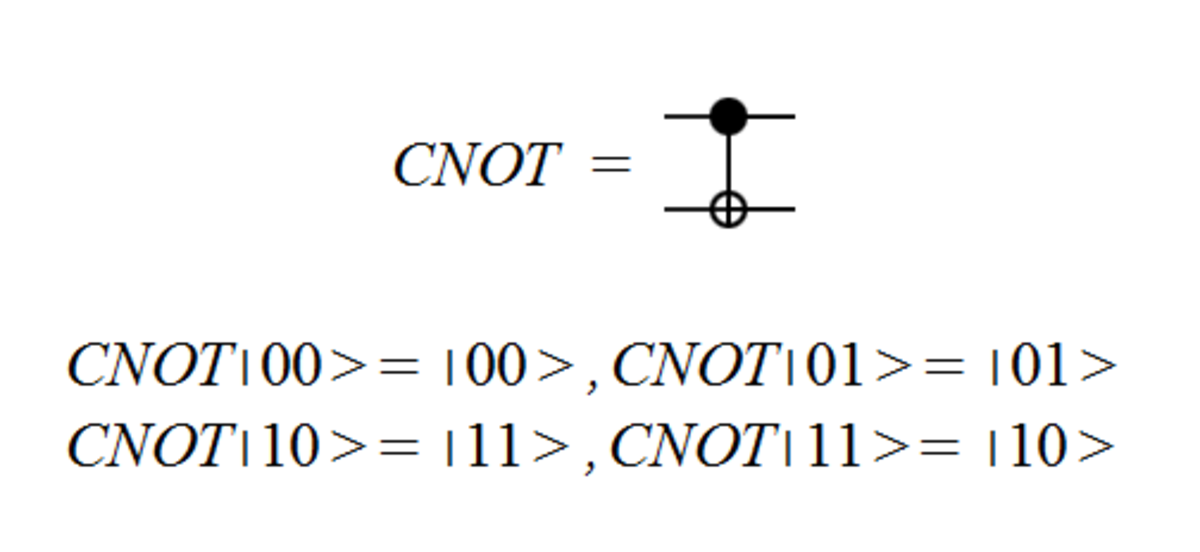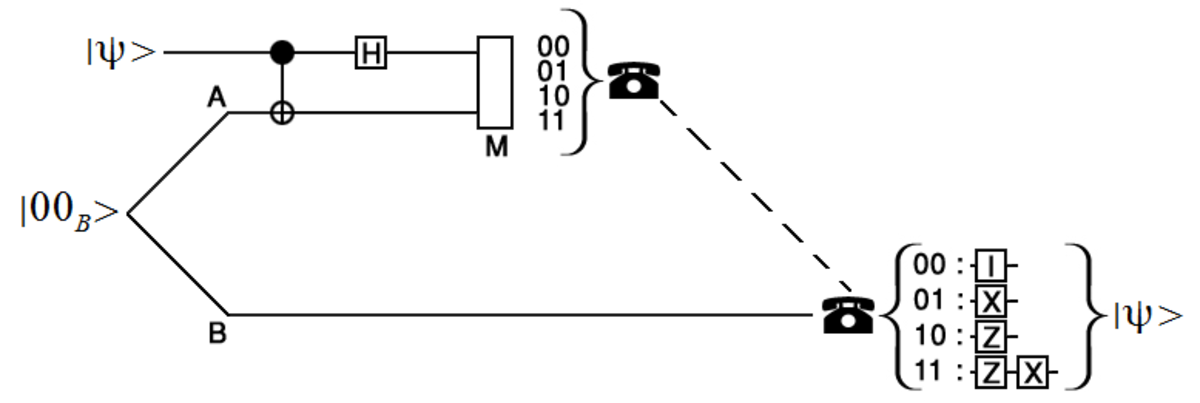# The Quantum Teleportation Protocol: How Does Quantum Teleportation Work?

Sam Brind holds a master's in physics with theoretical physics (MPhys) from the University of Manchester.

## What Is Quantum Teleportation?

Quantum teleportation is a technique for sending a quantum bit (qubit) over large distances. This doesn't initially sound very impressive, but it is a key technique within quantum computing. To solve this problem classically, a bit would just be copied and the copy then transmitted. However, an arbitrary qubit cannot be copied, this is a fundamental aspect of quantum computing known as the no-cloning theorem. Quantum teleportation is the main technique for sending qubits across large distances.

Before the protocol for implementing quantum teleportation can be understood, a brief introduction to qubits and quantum gates is required.

## Qubits

Unlike a classical bit, which is either a zero or a one, a qubit can be in both states at the same time. More formally, the qubit's state is fully described by a state vector that is a superposition of the two standard basis vectors, which represent the classical bits. A measurement of the qubit causes the state vector to collapse to a basis vector.

If there are two or more qubits, the space of possible state vectors is given by the tensor product of the individual qubit spaces. The mathematics of the tensor product is not needed in detail here. All we require are the standard basis vectors in a two qubit state space; these are given below.

The interaction of multiple qubits introduces the possibility of entanglement between qubits. Entanglement is one of the most interesting aspects of quantum mechanics and the main reason why a quantum computer behaves differently to a classical computer. The state vector of entangled qubits cannot be described by the tensor product of state vectors for the individual qubits. Essentially the qubits aren't independent but somehow they are linked together, even when separated by a large distance. When one of the qubits of an entangled qubit pair is measured the result of measuring the other qubit is determined.

The standard basis is the most common choice of basis but it isn't the only choice. An alternative two-qubit basis is the Bell basis {00B, 01B, 10B, 11B}. This basis is commonly used in quantum computing because all four Bell basis vectors are maximally entangled states.

## Quantum Gates

Analogous to how classical computers use circuits built out of logic gates, quantum circuits are built out of quantum gates. Gates can be represented by matrices, the result of applying the matrix is then given by multiplying the matrix by the state column vector. Equivalently, knowledge of the gates' effect on the basis vectors is sufficient to determine the result of applying the gate (as the state vector is a superposition of the basis vectors). Knowledge of five particular quantum gates is required for understanding the quantum teleportation protocol.

First, we will look at gates that act on a single qubit. The simplest of which is the identity gate (labelled as I). The identity gate leaves the basis vectors unchanged and is hence equivalent to "doing nothing".

The next gate is sometimes called the phase flip gate (Z). The phase flip gate leaves the zero basis vector unchanged but introduces a factor of minus one for the one basis vector.

Scroll to Continue

The next gate is the NOT gate (X). The NOT gate switches between the two basis vectors.

The final single qubit gate that is required is the Hadamard gate (H). This maps the basis vectors to superpositions of both basis vectors, as shown below.

Knowledge of a two-qubit gate, the controlled NOT gate (CNOT), is also required. The CNOT gate uses one of the input qubits as a control qubit. If the control qubit is set to one then the NOT gate is applied to the other input qubit.The circuit symbol for the CNOT gate and the effect of the CNOT gate on the two qubit basis states. The filled in black circle indicates the control qubit.

## Quantum Teleportation Protocol

The protocol for Alice to send a qubit, in an unknown arbitrary state, to Bob is as follows:

1. The bell basis state, 00B, is generated.
2. One of the qubits is given to Alice and the other qubit is given to Bob. Alice and Bob can then be spatially separated as much as they want.
3. Alice entangles the shared qubits with the qubit she wants to send. This is achieved by applying a CNOT gate to her two qubits followed by applying the Hadamard gate to the qubit she wants to send.
4. Alice performs a measurement, in the standard basis, of her two qubits.
5. Alice sends the result of her measurement to Bob over a classical communication channel. (Note: this introduces a time delay to prevent information from being transmitted instantaneously.)
6. Depending on the received result, Bob applies different single qubit gates to obtain the qubit Alice wanted to send.
7. Specifically: if 00 is received the identity gate is applied, if 01 is received the NOT gate is applied, if 10 is received the phase flip gate is applied and if 11 is received the NOT gate is applied followed by the application of the phase flip gate.A diagram that illustrates the quantum teleportation protocol. Solid lines indicate qubit channels and a dashed line represents a classical communication channel.

## Mathematical Proof

Initially Alice and Bob share the qubits of the bell basis state 00B and Alice also has a qubit she wants to send. The total state of these three qubits is:

Alice then applies the CNOT gate to the two qubits in her possession, this changes the state to:

Alice then applies the Hadamard gate to the qubit she wishes to send, this changes the state to:

The previous state can be mathematically rearranged into an equivalent expression. This alternate form clearly shows the entanglement of Bob's qubit with Alice's two qubits.

Alice then measures her two qubits in the standard basis. The result will be one of the four possible bit strings {00, 01, 10, 11}. The act of measurement causes the state of Bob's qubit to collapse to one of four possible values. The possible outcomes are listed below.

## Has This Actually Been Experimentally Realised?

The principle of quantum teleportation was physically demonstrated only a few years after the protocol was theoretically developed . Since then the distance of teleportation has been gradually increased. The current record is teleportation over a distance of 143 km (between two of the Canary Islands) . Further development of effective quantum teleportation methods is crucial for building networks of quantum computers, such as a future "quantum internet".

A final point to note is that the state of the qubit has been sent to another qubit, ie. only information has been sent, not the physical qubit. This is contrary to the popular picture of teleportation popularized by science fiction.

 D. Boschi et al., Experimental Realization of Teleporting an Unknown Pure Quantum State via Dual Classical and Einstein-Podolski-Rosen Channels, arXiv, 1997, URL: https://arxiv.org/pdf/quant-ph/9710013.pdf

 X. Ma et al., Quantum teleportation using active feed-forward between two Canary Islands, arXiv, 2012, URL: https://arxiv.org/pdf/1205.3909.pdf